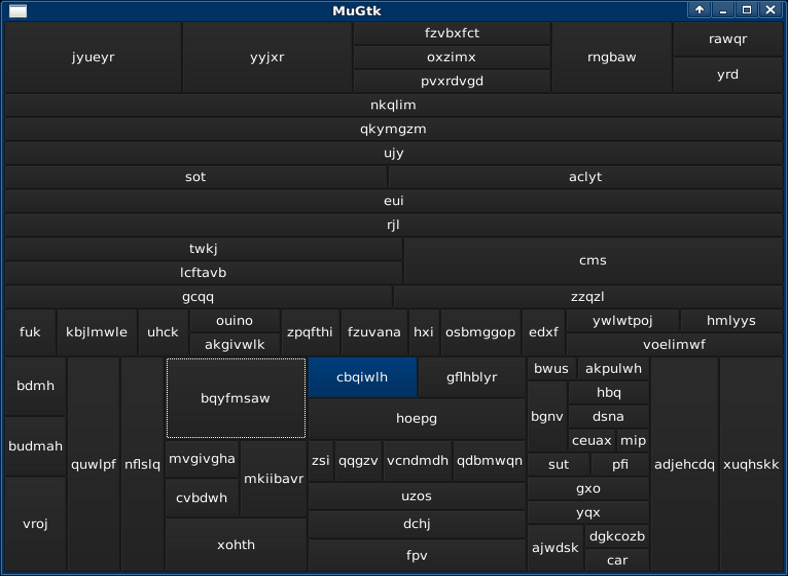# mathr / blog / #module Main (main) where

import System.Environment (getArgs)
import System.Random (randomIO, randomRIO)
import Graphics.UI.Gtk

I was slightly bored and insomniac and pining for the days of AmigaE/EasyGUI (no, not the later imitations), so I thought about making a simple wrapper around GTK to replicate some of that ease of use in Haskell. I didn't get very far before I was distracted by shiny ideas...

type W = IO Widget

layout :: BoxClass b => (Bool -> Int -> IO b) -> [W] -> W
layout box ws = do
b <- box False 0
mapM_ (>>= \w -> boxPackStart b w PackGrow 0) ws
return $toWidget b rows, cols :: [W] -> W rows = layout vBoxNew cols = layout hBoxNew button :: String -> IO () -> W button name act = do b <- buttonNewWithLabel name _ <- b on buttonActivated$ act
return $toWidget b ...like interpreting values to turn them into real life GUIs... data GUI = Button String | Rows [GUI] | Cols [GUI] interpret :: GUI -> W interpret (Button g) = button g (putStrLn g) interpret (Rows g) = rows (map interpret g) interpret (Cols g) = cols (map interpret g) ...and generating random values and GUIs within a given size limit: randomGUI :: Int -> IO GUI randomGUI n | n < 2 = do l <- randomRIO (3, 8) s <- replicateM l$ randomRIO ('a', 'z')
return $Button s | otherwise = do d <- randomRIO (1, n - 1) w <- randomIO l <- randomGUI$     d
r <- randomGUI $n - d - 2 -- termination assured by (n - d - 2) + d < n return$ if w then Rows [l, r] else Cols [l, r]

Which just requires a minimal GTK driver to display the top-level widget...

gui :: W -> IO ()
gui wc = do
_ <- initGUI
w <- windowNew
c <- wc
set w [ containerChild := c ]
_ <- onDestroy w mainQuit
widgetShowAll w
mainGUI

...and a braindead command-line argument "parser" to finish the whole program off:

main :: IO ()
main = gui . interpret =<< randomGUI =<< fmap (read . head) getArgs

Full source code without the HTML entities: RandomGUI.hs